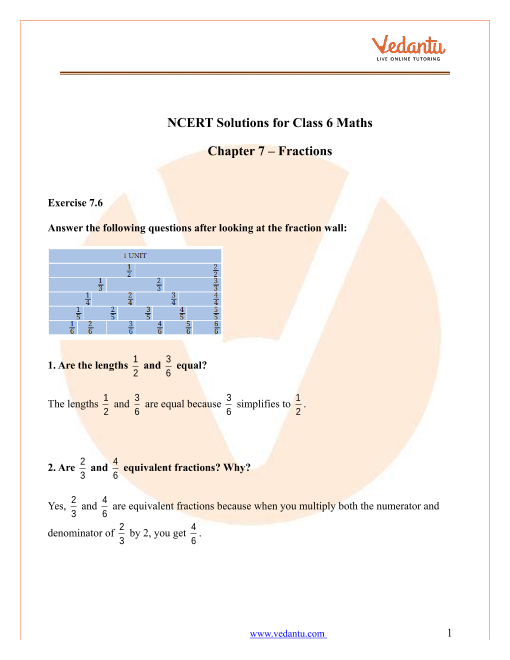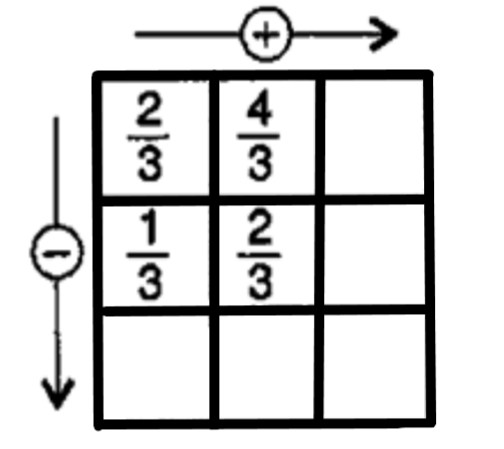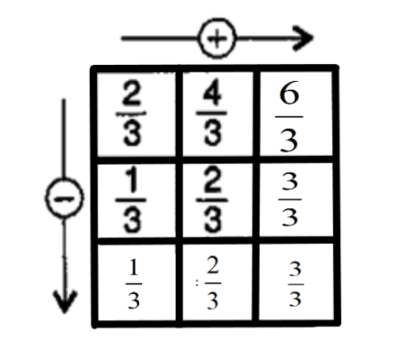# NCERT Solutions for Class 6 Maths Chapter 7 Fractions (Ex 7.6) Exercise 7.6

## NCERT Solutions for Class 6 Maths Chapter 7 Fractions (Ex 7.6) Exercise 7.6

Free PDF download of NCERT Solutions for Class 6 Maths Chapter 7 Exercise 7.6 (Ex 7.6) and all chapter exercises at one place prepared by an expert teacher as per NCERT (CBSE) books guidelines. Class 6 Maths Chapter 7 Fractions Exercise 7.6 Questions with Solutions to help you to revise complete Syllabus and Score More marks. Register and get all exercise solutions in your emails.

Every NCERT Solution is provided to make the study simple and interesting on Vedantu. Subjects like Science, Maths, English,Hindi will become easy to study if you have access to NCERT Solution for Class 6 Science , Maths solutions and solutions of other subjects.

Do you need help with your Homework? Are you preparing for Exams?
Study without Internet (Offline)Book your Free Demo session
Get a flavour of LIVE classes here at Vedantu## NCERT Solutions for Class 6 Maths Chapter 7 Fractions Exercise 7.6

Refer to page 17 for Exercise 7.6 in the PDF

1. Solve the following:

1. $\dfrac{2}{3} + \dfrac{1}{7}$

Ans: Take the LCM of denominator to find the above sum.

The LCM of 7 and 3 is 21.

$\dfrac{2}{3} + \dfrac{1}{7} = \dfrac{{2 \times 7 + 1 \times 3}}{{21}}$

$\dfrac{2}{3} + \dfrac{1}{7} = \dfrac{{14 + 3}}{{21}}$

$\dfrac{2}{3} + \dfrac{1}{7} = \dfrac{{17}}{{21}}$

Thus, the sum is $\dfrac{{17}}{{21}}$.

1. $\dfrac{3}{{10}} + \dfrac{7}{{15}}$

Ans: Take the LCM of denominator to find the above sum.

The LCM of 10 and 15 is 30.

$\dfrac{3}{{10}} + \dfrac{7}{{15}} = \dfrac{{3 \times 3 + 7 \times 2}}{{30}}$

$\dfrac{3}{{10}} + \dfrac{7}{{15}} = \dfrac{{9 + 14}}{{30}}$

$\dfrac{3}{{10}} + \dfrac{7}{{15}} = \dfrac{{23}}{{30}}$

Thus, the sum is $\dfrac{{23}}{{30}}$.

1. $\dfrac{4}{9} + \dfrac{2}{7}$

Ans: Take the LCM of denominator to find the above sum.

The LCM of 9 and 7 is 63.

$\dfrac{4}{9} + \dfrac{2}{7} = \dfrac{{4 \times 7 + 2 \times 9}}{{63}}$

$\dfrac{4}{9} + \dfrac{2}{7} = \dfrac{{28 + 18}}{{63}}$

$\dfrac{4}{9} + \dfrac{2}{7} = \dfrac{{46}}{{63}}$

Thus, the sum is $\dfrac{{46}}{{63}}$.

1. $\dfrac{5}{7} + \dfrac{1}{3}$

Ans: Take the LCM of denominator to find the above sum.

The LCM of 7 and 3 is 21.

$\dfrac{5}{7} + \dfrac{1}{3} = \dfrac{{5 \times 3 + 1 \times 7}}{{21}}$

$\dfrac{5}{7} + \dfrac{1}{3} = \dfrac{{15 + 7}}{{21}}$

$\dfrac{5}{7} + \dfrac{1}{3} = \dfrac{{22}}{{21}}$

Thus, the sum is $\dfrac{{22}}{{21}}$.

1. $\dfrac{2}{5} + \dfrac{1}{6}$

Ans: Take the LCM of denominator to find the above sum.

The LCM of 5 and 6 is 30.

$\dfrac{2}{5} + \dfrac{1}{6} = \dfrac{{2 \times 6 + 1 \times 5}}{{30}}$

$\dfrac{2}{5} + \dfrac{1}{6} = \dfrac{{12 + 5}}{{30}}$

$\dfrac{2}{5} + \dfrac{1}{6} = \dfrac{{17}}{{30}}$

Thus, the sum is $\dfrac{{17}}{{30}}$.

1. $\dfrac{4}{5} + \dfrac{2}{3}$

Ans: Take the LCM of denominator to find the above sum.

The LCM of 5 and 3 is 15.

$\dfrac{4}{5} + \dfrac{2}{3} = \dfrac{{4 \times 3 + 2 \times 5}}{{15}}$

$\dfrac{4}{5} + \dfrac{2}{3} = \dfrac{{12 + 10}}{{15}}$

$\dfrac{4}{5} + \dfrac{2}{3} = \dfrac{{22}}{{15}}$

Thus, the sum is $\dfrac{{22}}{{15}}$.

1. $\dfrac{3}{4} - \dfrac{1}{3}$

Ans: Take the LCM of denominator to find the above difference.

The LCM of 4 and 3 is 12.

$\dfrac{3}{4} - \dfrac{1}{3} = \dfrac{{3 \times 3 - 1 \times 4}}{{12}}$

$\dfrac{3}{4} - \dfrac{1}{3} = \dfrac{{9 - 4}}{{12}}$

$\dfrac{3}{4} - \dfrac{1}{3} = \dfrac{5}{{12}}$

Thus, the difference is $\dfrac{5}{{12}}$.

1. $\dfrac{5}{6} - \dfrac{1}{3}$

Ans: Take the LCM of denominator to find the above difference.

The LCM of 6 and 3 is 6.

$\dfrac{5}{6} - \dfrac{1}{3} = \dfrac{{5 \times 1 - 1 \times 2}}{6}$

$\dfrac{5}{6} - \dfrac{1}{3} = \dfrac{{5 - 2}}{6}$

$\dfrac{5}{6} - \dfrac{1}{3} = \dfrac{3}{6}$

Thus, the difference is $\dfrac{3}{6}$.

1. $\dfrac{2}{3} + \dfrac{3}{4} + \dfrac{1}{2}$

Ans: Take the LCM of denominator to find the above sum.

The LCM of 3, 4, and 2 is 12.

$\dfrac{2}{3} + \dfrac{3}{4} + \dfrac{1}{2} = \dfrac{{2 \times 4 + 3 \times 3 + 1 \times 6}}{{12}}$

$\dfrac{2}{3} + \dfrac{3}{4} + \dfrac{1}{2} = \dfrac{{8 + 9 + 6}}{{12}}$

$\dfrac{2}{3} + \dfrac{3}{4} + \dfrac{1}{2} = \dfrac{{23}}{{12}}$

Thus, the sum is $\dfrac{{23}}{{12}}$.

1. $\dfrac{1}{2} + \dfrac{1}{3} + \dfrac{1}{6}$

Ans: Take the LCM of denominator to find the above sum.

The LCM of 2, 3, and 6 is 6.

$\dfrac{1}{2} + \dfrac{1}{3} + \dfrac{1}{6} = \dfrac{{1 \times 3 + 1 \times 2 + 1 \times 1}}{6}$

$\dfrac{1}{2} + \dfrac{1}{3} + \dfrac{1}{6} = \dfrac{{3 + 2 + 1}}{6}$

$\dfrac{1}{2} + \dfrac{1}{3} + \dfrac{1}{6} = \dfrac{6}{6}$

Thus, the sum is $\dfrac{6}{6}$.

1. $1\dfrac{1}{3} + 3\dfrac{2}{3}$

Ans: Covert the mixed fraction into proper fractions.

$1\dfrac{1}{3} + 3\dfrac{2}{3} = \dfrac{4}{3} + \dfrac{{11}}{3}$

Take the LCM of denominator to find the above sum.

$\dfrac{4}{3} + \dfrac{{11}}{3} = \dfrac{{4 + 11}}{3}$

$\dfrac{4}{3} + \dfrac{{11}}{3} = \dfrac{{15}}{3}$

Thus, the sum is $\dfrac{{15}}{3}$.

1. $4\dfrac{2}{3} + 3\dfrac{1}{4}$

Ans: Covert the mixed fraction into proper fractions.

$4\dfrac{2}{3} + 3\dfrac{1}{4} = \dfrac{{14}}{3} + \dfrac{{13}}{4}$

Take the LCM of denominator to find the above sum.

The LCM of 3 and 4 is 12.

$\dfrac{{14}}{3} + \dfrac{{13}}{4} = \dfrac{{14 \times 4 + 13 \times 3}}{{12}}$

$\dfrac{{14}}{3} + \dfrac{{13}}{4} = \dfrac{{56 + 39}}{{12}}$

$\dfrac{{14}}{3} + \dfrac{{13}}{4} = \dfrac{{95}}{{12}}$

Thus, the sum is $\dfrac{{95}}{{12}}$.

1. $\dfrac{{16}}{5} - \dfrac{7}{5}$

Ans: Take the LCM of denominator to find the above difference.

$\dfrac{{16}}{5} - \dfrac{7}{5} = \dfrac{{16 - 7}}{5}$

$\dfrac{{16}}{5} - \dfrac{7}{5} = \dfrac{9}{5}$

Thus, the difference is $\dfrac{9}{5}$.

1. $\dfrac{4}{3} - \dfrac{1}{2}$

Ans: Take the LCM of denominator to find the above difference.

The LCM of 3 and 2 is 6.

$\dfrac{4}{3} - \dfrac{1}{2} = \dfrac{{4 \times 2 - 1 \times 3}}{6}$

$\dfrac{4}{3} - \dfrac{1}{2} = \dfrac{{8 - 3}}{6}$

$\dfrac{4}{3} - \dfrac{1}{2} = \dfrac{5}{6}$

Thus, the difference is $\dfrac{5}{6}$.

2. Sarika bought $\dfrac{2}{5}$ meter of ribbon and Lalita $\dfrac{3}{4}$ meter of ribbon. What is the total length of the ribbon they bought?

Ans: The total amount of ribbon bought by Sarita is $\dfrac{2}{5}$ meter and the ribbon bought by Lalita is $\dfrac{3}{4}$ meter.

To find the total length of the ribbon bought, we will add both the measurements.

${\text{Total length}} = \dfrac{2}{5} + \dfrac{3}{4}$

Take the LCM of  denominator to find the above sum.

The LCM of 4 and 5 is 20.

${\text{Total length}} = \dfrac{{2 \times 4 + 5 \times 3}}{{20}}$

${\text{Total length}} = \dfrac{{8 + 15}}{{20}}$

${\text{Total length}} = \dfrac{{23}}{{20}}$

Hence, the total length of ribbon bought is $\dfrac{{23}}{{20}}$ meter.

3. Naina was given $1\dfrac{1}{2}$ piece of cake and Najma was given $1\dfrac{1}{3}$ piece of cake. Find the total amount of cake given to both of them.

Ans: Naina got $1\dfrac{1}{2}$piece of cake, that is, $\dfrac{3}{2}$ piece of cake.

Najma bought $1\dfrac{1}{3}$ piece of cake, that is, $\dfrac{4}{3}$ piece of cake.

So, add the fractions to get the total amount of cake that was given to Naina and Najma.

${\text{Total cake}} = \dfrac{3}{2} + \dfrac{4}{3}$.

To find the above addition, we will take the LCM of the denominators and solve.

The LCM of 2 and 3 is 6.

${\text{Total cake}} = \dfrac{{3 \times 3 + 4 \times 2}}{6}$

${\text{Total cake}} = \dfrac{{9 + 8}}{6}$

${\text{Total cake}} = \dfrac{{17}}{6}$

Hence, the total amount of cake they both have is $\dfrac{{17}}{6}$.

4. Fill in the boxes.

1. $\boxed{} - \dfrac{5}{8} = \dfrac{1}{4}$

Ans:Add $\dfrac{5}{8}$ to both sides and take LCM of 4 and 8.

The LCM of 4 and 8 is 32.

$\dfrac{1}{4} + \dfrac{5}{8} = \dfrac{{1 \times 2 + 1 \times 5}}{8}$

$\dfrac{1}{4} + \dfrac{5}{8} = \dfrac{{2 + 5}}{8}$

$\dfrac{1}{4} + \dfrac{5}{8} = \dfrac{7}{8}$

Hence, the missing fraction is $\dfrac{7}{8}$.

1. $\boxed{} - \dfrac{1}{5} = \dfrac{1}{2}$

Ans:Add $\dfrac{1}{5}$ to both sides and take LCM of 2 and 5.

The LCM of 2 and 5 is 10.

$\dfrac{1}{5} + \dfrac{1}{2} = \dfrac{{1 \times 2 + 1 \times 5}}{{10}}$

$\dfrac{1}{5} + \dfrac{1}{2} = \dfrac{{2 + 5}}{{10}}$

$\dfrac{1}{5} + \dfrac{1}{2} = \dfrac{7}{{10}}$

Hence, the missing fraction is $\dfrac{7}{{10}}$.

1. $\dfrac{1}{2} - \boxed{} = \dfrac{1}{6}$

Ans: Subtract $\dfrac{1}{6}$ from both sides.

Take LCM of 2 and 6.

The LCM of 2 and 6 is 6.

$\dfrac{1}{2} - \dfrac{1}{6} = \dfrac{{1 \times 3 - 1 \times 1}}{6}$

$\dfrac{1}{2} - \dfrac{1}{6} = \dfrac{{3 - 1}}{6}$

$\dfrac{1}{2} - \dfrac{1}{6} = \dfrac{2}{6}$

Hence, the missing fraction is $\dfrac{2}{6}$.

1.Ans: To complete the given box, add the rows and subtract the column simultaneously.

Add $\dfrac{2}{3}$and $\dfrac{4}{3}$.

$\dfrac{2}{3} + \dfrac{4}{3} = \dfrac{{2 + 4}}{3}$

$\dfrac{2}{3} + \dfrac{4}{3} = \dfrac{6}{3}$

Add $\dfrac{1}{3}$ and $\dfrac{2}{3}$.

$\dfrac{1}{3} + \dfrac{2}{3} = \dfrac{{1 + 2}}{3}$

$\dfrac{1}{3} + \dfrac{2}{3} = \dfrac{3}{3}$

Subtract $\dfrac{2}{3}$ and $\dfrac{1}{3}$.

$\dfrac{2}{3} - \dfrac{1}{3} = \dfrac{{2 - 1}}{3}$

$\dfrac{2}{3} - \dfrac{1}{3} = \dfrac{1}{3}$

Subtract $\dfrac{4}{3}$ and $\dfrac{2}{3}$.

$\dfrac{4}{3} - \dfrac{2}{3} = \dfrac{{4 - 2}}{3}$

$\dfrac{4}{3} - \dfrac{2}{3} = \dfrac{2}{3}$

Now, complete the third column. To do so, subtract $\dfrac{6}{3}$ and $\dfrac{3}{3}$.

$\dfrac{6}{3} - \dfrac{3}{3} = \dfrac{{6 - 3}}{3}$

$\dfrac{6}{3} - \dfrac{3}{3} = \dfrac{3}{3}$

Therefore, the complete box is as follows.6. A piece of wire $\dfrac{7}{8}$ meter long broke into two pieces. One piece was $\dfrac{1}{4}$ meter long. How long is the other piece?

Ans: The total length of the wire is $\dfrac{7}{8}$ meter. The length of the first piece is $\dfrac{1}{4}$ meter.

The length of remaining part will be,

${\text{Remaining part}} = \dfrac{7}{8} - \dfrac{1}{4}$

Take the LCM of denominator to find the above difference.

The LCM of 4 and 8 is 32.

${\text{Remaining part}} = \dfrac{{7 - 2}}{8}$

${\text{Remaining part}} = \dfrac{5}{8}$

Therefore, the length of the remaining part  $\dfrac{5}{8}$ meter.

7. Nandini house is $\dfrac{9}{{10}}$ km from her school. She walked some distance and then took as bus for $\dfrac{1}{2}$ km to reach the school. How far did she walk?

Ans: The total distance between school and house is $\dfrac{9}{{10}}$ km and the distance covered by bus is $\dfrac{1}{2}$ km.

The distance she has to walk can be found out by finding the difference between the distance covered by bus and distance between school and house.

${\text{Remaining distance}} = \dfrac{9}{{10}} - \dfrac{1}{2}$

Take the LCM of denominator to find the above difference.

The LCM of 10 and 2 is 10.

${\text{Remaining distance}} = \dfrac{{9 - 5}}{{10}}$

${\text{Remaining distance}} = \dfrac{4}{{10}}$

Thus, the remaining distance is $\dfrac{4}{{10}}$ km.

8. Ahsa and Samuel have bookshelves of the same size partly filled with books. Asha’s shelf is${\dfrac{5}{6}^{{\text{th}}}}$full and Samuel’s shelf is ${\dfrac{2}{5}^{{\text{th}}}}$ full. Whose bookshelf is filled with more books? By what fraction?

Ans: To solve this, we will make the denominator of both the fraction equal.

To do so, multiply the numerator and denominator of $\dfrac{5}{6}$ and $\dfrac{2}{5}$ by 5 and 6 respectively.

$\dfrac{{5 \times 5}}{{6 \times 5}} = \dfrac{{25}}{{30}}$

$\dfrac{{2 \times 6}}{{5 \times 6}} = \dfrac{{12}}{{30}}$

Thus, $\dfrac{{25}}{{30}}\boxed > \dfrac{{12}}{{30}}$.

Therefore, $\dfrac{5}{6}\boxed > \dfrac{2}{5}$. Asha’s bookshelf is more covered than Samuel.

The difference between the books they both have is,

${\text{Difference}} = \dfrac{{25}}{{30}} - \dfrac{{12}}{{30}}$

${\text{Difference}} = \dfrac{{25 - 12}}{{30}}$

${\text{Difference}} = \dfrac{{13}}{{30}}$

Thus, Asha’s book shelf is covered with more books and she has $\dfrac{{13}}{{30}}$ more books in her shelf.

9. Jaidev takes $2\dfrac{1}{5}$ minutes to walk across the school ground. Rahul takes $\dfrac{7}{4}$ minutes to do same. Who takes less time and by what fraction?

Ans: The total time taken by Jaidev is$2\dfrac{1}{5}$ minutes.

On converting $2\dfrac{1}{5}$ we get,

$2\dfrac{1}{5} = \dfrac{{11}}{5}{\text{minutes}}$

The total time taken by Rahul is $\dfrac{7}{4}$ minutes.

To find who takes less time we will find the difference between time taken by Jaidev and Rahul.

$\dfrac{{11}}{5} - \dfrac{7}{4}$

Take LCM of 5 and 4. Thus, the LCM of 5 and 4 is 20.

${\text{Difference}} = \dfrac{{11 \times 4 - 7 \times 5}}{{20}}$

${\text{Difference}} = \dfrac{{44 - 35}}{{20}}$

${\text{Difference}} = \dfrac{9}{{20}}\,{\text{minutes}}$

Thus, Rahul takes less time, which is $\dfrac{9}{{20}}$ minutes.

## NCERT Solutions for Class 6 Maths Chapter 7 Fractions Exercise 7.6

Opting for the NCERT solutions for Ex 7.6 Class 6 Maths is considered as the best option for the CBSE students when it comes to exam preparation. This chapter consists of many exercises. Out of which we have provided the Exercise 7.6 Class 6 Maths NCERT solutions on this page in PDF format. You can download this solution as per your convenience or you can study it directly from our website/ app online.

Vedantu in-house subject matter experts have solved the problems/ questions from the exercise with the utmost care and by following all the guidelines by CBSE. Class 6 students who are thorough with all the concepts from the Maths textbook and quite well-versed with all the problems from the exercises given in it, then any student can easily score the highest possible marks in the final exam. With the help of this Class 6 Maths Chapter 7 Exercise 7.6 solutions, students can easily understand the pattern of questions that can be asked in the exam from this chapter and also learn the marks weightage of the chapter. So that they can prepare themselves accordingly for the final exam.

Besides these NCERT solutions for Class 6 Maths Chapter 7 Exercise 7.6, there are plenty of exercises in this chapter which contain innumerable questions as well. All these questions are solved/answered by our in-house subject experts as mentioned earlier. Hence all of these are bound to be of superior quality and anyone can refer to these during the time of exam preparation. In order to score the best possible marks in the class, it is really important to understand all the concepts of the textbooks and solve the problems from the exercises given next to it.

Do not delay any more. Download the NCERT solutions for Class 6 Maths Chapter 7 Exercise 7.6 from Vedantu website now for better exam preparation. If you have the Vedantu app in your phone, you can download the same through the app as well. The best part of these solutions is these can be accessed both online and offline as well.SHARETWEETSHARESUBSCRIBE